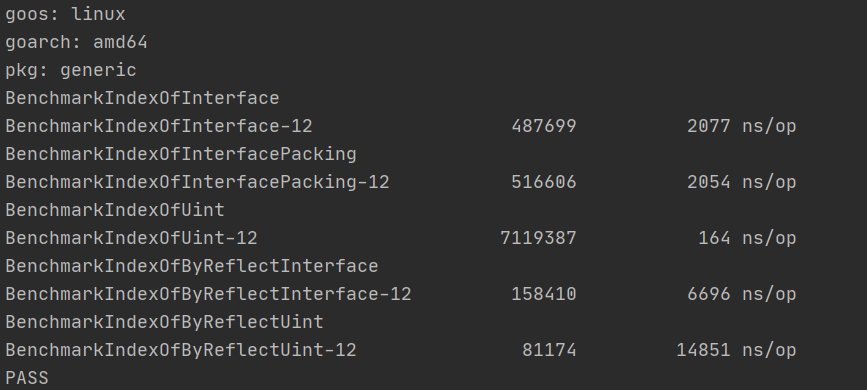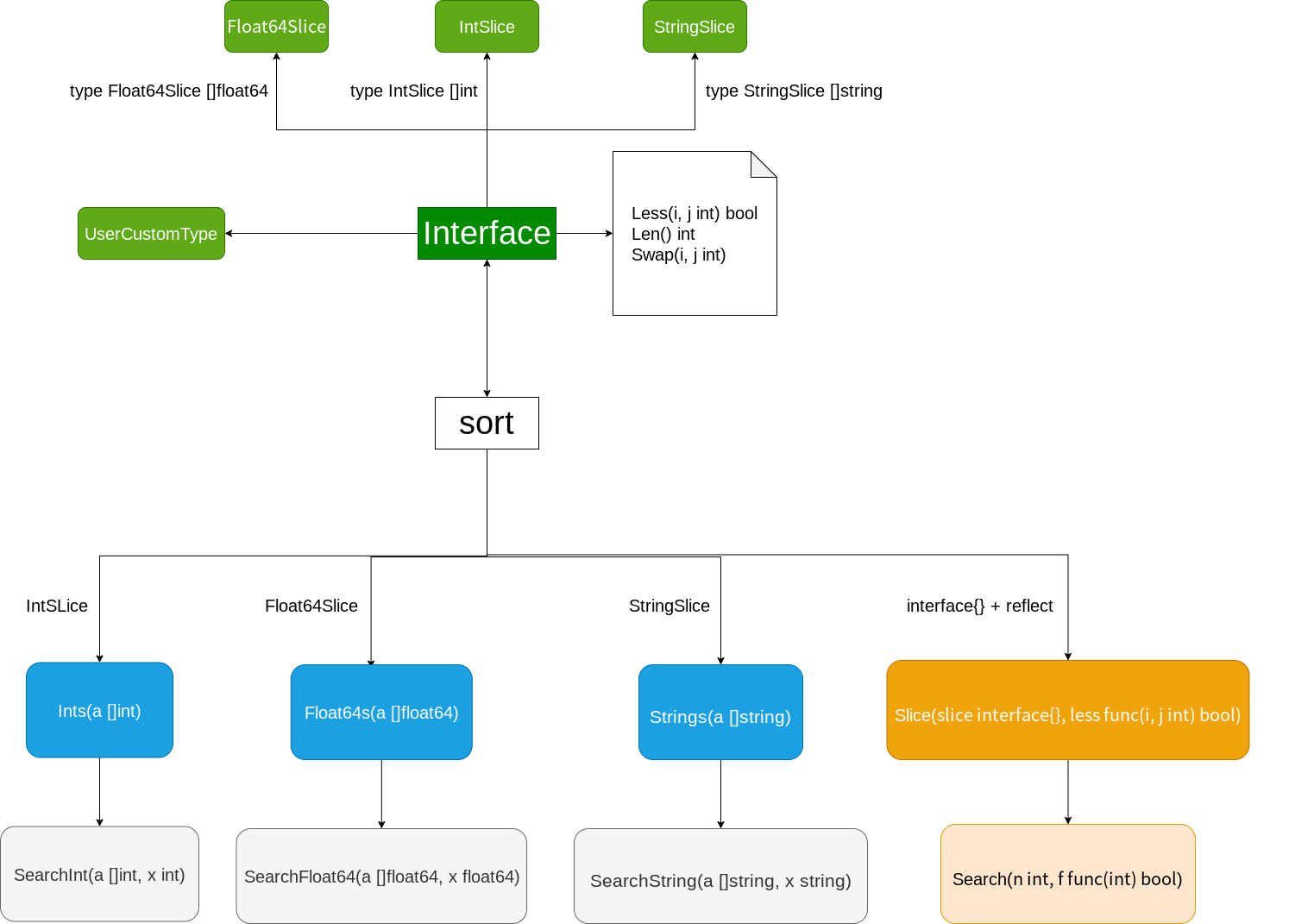# golang拾遗：为什么我们需要泛型

## 没有泛型的世界

### 暴力穷举

float a = logf(2.0f);
double b = log(2.0);

typedef struct {
int *data;
unsigned int max_size;
} IntQueue;

typedef struct {
double *data;
unsigned int max_size;
} DoubleQueue;

IntQueue* NewIntQueue(unsigned int size)
{
IntQueue* q = (IntQueue*)malloc(sizeof(IntQueue));
if (q == NULL) {
return NULL;
}
q->max_size = size;
q->data = (int*)malloc(size * sizeof(int));
return q;
}

DoubleQueue* NewDoubleQueue(unsigned int size)
{
DoubleQueue* q = (DoubleQueue*)malloc(sizeof(DoubleQueue));
if (q == NULL) {
return NULL;
}
q->max_size = size;
q->data = (double*)malloc(size * sizeof(double));
return q;
}


• 严重违反DRY（don't repeat yourself），数据结构的修改和扩展极其困难
• 复制粘贴修改中可能会出现低级的人力错误，并且耗费精力
• 最关键的一点，我们不可能针对所有类型去写出特定的算法，因为这些类型的数量少则5,6种，多则上不封顶。

• 保证了类型安全，任何类型问题都能在编译期暴露
• 更灵活，对于某些特定类型我们还可以做出非常细致的优化工作（比如对于bool类型我们可以使用unsigned int这个一般来说4字节大小的类型存放32个bool值，而不是用32个bool变量消耗32字节内存）

### 依靠通用引用类型

void *ptr = NULL;
ptr = (void*)"hello";
int a = 100;
ptr = (void*)&a;


c语言允许非函数指针的数据类型指针转换为void *，因此我们可以用它来囊括几乎所有的数据（函数除外）。

typedef struct {
void *data;
unsigned int max_size;
} Queue;

Queue* NewQueue(unsigned int size)
{
Queue* q = (Queue*)malloc(sizeof(Queue));
if (q == NULL) {
return NULL;
}
q->max_size = size;
q->data = // 这里填什么呢？
}


typedef struct {
unsigned int max_size;
unsigned int current;
void **data;
} Queue;

Queue* NewQueue(unsigned int size)
{
Queue* q = (Queue*)malloc(sizeof(Queue));
if (q == NULL) {
return NULL;
}
q->max_size = size;
q->size = 0;
q->data = (void **)malloc(size*sizeof(void*));
}

bool QueuePush(Queue* q, void* value)
{
if (q == NULL || value == NULL || q->current == q->max_size-1) {
return false;
}

q->data[q->current++] = value;
return true;
}


It works! 但是我们需要队列中的类型有特定操作呢？把操作抽象形成函数再传递给队列的方法就行了，可以参考c的qsort和bsearch：

#include <stdlib.h>

void qsort(void *base, size_t nmemb, size_t size,
int (*compar)(const void *, const void *));

void *bsearch(const void *key, const void *base,
size_t nmemb, size_t size,
int (*compar)(const void *, const void *));


typedef struct node {
int val;
struct node *next;
} node_t;

void enqueue(node_t **head, int val) {
node_t *new_node = malloc(sizeof(node_t));
if (!new_node) return;

new_node->val = val;

}


• 首先是类型擦除的同时没有任何类型检测的手段，因此类型安全无从保证，比如存进去的可以是int，取出来的时候你可以转换成char*，程序不会给出任何警告，等你准备从这个char*里取出某个位置上的字符的时候就会引发未定义行为，从而出现许许多多奇形怪状的bug
• 只能存指针类型
• 如何确定队列里存储数据的所有权？交给队列管理会增加队列实现的复杂性，不交给队列管理就需要手动追踪N个对象的生命周期，心智负担很沉重，并且如果我们是存入的局部变量的指针，那么交给队列管理就一定会导致free出现未定义行为，从代码层面我们是几乎不能区分一个指针是不是真的指向了堆上的内容的
• 依旧不能避免书写类型代码，首先使用数据时要从void*转换为对应类型，其次我们需要书写如qsort例子里那样的帮助函数。

### 动态类型语言的特例

int a = 0;
const char *str = "hello generic";
double values[] = {1., 2., 3.};


name = 'apocelipes'
name = 12345


name究竟是什么类型的变量？不知道，因为name实际上可以赋值任意的数据，我们只能在运行时的某个点做类型检测，然后断言name是xxx类型的，然而过了这个时间点之后name还可以赋值一个完全不同类型的数据。

class Queue:
def __init__(self):
self.data = []

def push(self, value):
self.data.append()

def pop(self):
self.data.pop()

def take(self, index):
return self.data[index]


def transform(self):
for i in range(len(self.data)):
self.data[i] = self.data[i].upper()


• 写出了类型相关的代码，和我们本意上想要实现类型无关的代码结构相冲突
• 限定了算法只能由几种数据类型使用，但事实上有无限多的类型可以实现upper方法，然而我们不能在类型检查里一一列举他们，从而导致了我们的通用算法变为了限定算法。

## 动静结合

### 使用interface模拟泛型

interface可以接受任何满足要求的类型的数据，并且具有运行时的类型检查。双保险很大程度上提升了代码的安全性。

package list // import "container/list"

Package list implements a doubly linked list.

To iterate over a list (where l is a *List):

for e := l.Front(); e != nil; e = e.Next() {
// do something with e.Value
}

type Element struct{ ... }
type List struct{ ... }
func New() *List

type Element struct {

// The value stored with this element.
Value interface{}
// Has unexported fields.
}
Element is an element of a linked list.

func (e *Element) Next() *Element
func (e *Element) Prev() *Element

type List struct {
// Has unexported fields.
}
List represents a doubly linked list. The zero value for List is an empty

func New() *List
func (l *List) Back() *Element
func (l *List) Front() *Element
func (l *List) Init() *List
func (l *List) InsertAfter(v interface{}, mark *Element) *Element
func (l *List) InsertBefore(v interface{}, mark *Element) *Element
func (l *List) Len() int
func (l *List) MoveAfter(e, mark *Element)
func (l *List) MoveBefore(e, mark *Element)
func (l *List) MoveToBack(e *Element)
func (l *List) MoveToFront(e *Element)
func (l *List) PushBack(v interface{}) *Element
func (l *List) PushBackList(other *List)
...


func IndexOfInterface(arr []interface{}, value interface{}) int {
for i, v := range arr {
if v == value {
return i
}
}

return -1
}


### interface会进行严格的类型检查

func ExampleIndexOfInterface() {
arr := []interface{}{uint(1),uint(2),uint(3),uint(4),uint(5)}
fmt.Println(IndexOfInterface(arr, 5))
fmt.Println(IndexOfInterface(arr, uint(5)))
// Output:
// -1
// 4
}


type Comparator interface {
Compare(v interface{}) bool
}

func IndexOfComparator(arr []Comparator, value Comparator) int {
for i,v := range arr {
if v.Compare(value) {
return i
}
}
return -1
}


### 内置类型何去何从

cannot use arr (type []interface {}) as type []Comparator in argument to IndexOfComparator


type MyUint uint

func (u MyUint) Compare(v interface{}) bool {
value := v.(MyUint)
return u == value
}

arr2 := []Comparator{MyUint(1),MyUint(2),MyUint(3),MyUint(4),MyUint(5)}
fmt.Println(IndexOfComparator(arr2, MyUint(5)))


### 性能陷阱

func IndexOfByReflect(arr interface{}, value interface{}) int {
arrValue := reflect.ValueOf(arr)
length := arrValue.Len()
for i := 0; i < length; i++ {
if arrValue.Index(i).Interface() == value {
return i
}
}
return -1
}

func IndexOfInterface(arr []interface{}, value interface{}) int {
for i, v := range arr {
if v == value {
return i
}
}

return -1
}

func IndexOfInterfacePacking(value interface{}, arr ...interface{}) int {
for i, v := range arr {
if v == value {
return i
}
}

return -1
}


const ArrLength = 500
var _arr []interface{}
var _uintArr []uint

func init() {
_arr = make([]interface{}, ArrLength)
_uintArr = make([]uint, ArrLength)
for i := 0; i < ArrLength - 1; i++ {
_uintArr[i] = uint(rand.Int() % 10 + 2)
_arr[i] = _uintArr[i]
}
_arr[ArrLength - 1] = uint(1)
_uintArr[ArrLength - 1] = uint(1)
}

func BenchmarkIndexOfInterface(b *testing.B) {
for i := 0; i < b.N; i++ {
IndexOfInterface(_arr, uint(1))
}
}

func BenchmarkIndexOfInterfacePacking(b *testing.B) {
for i := 0; i < b.N; i++ {
IndexOfInterfacePacking(uint(1), _arr...)
}
}

func indexOfUint(arr []uint, value uint) int {
for i,v := range arr {
if v == value {
return i
}
}

return -1
}

func BenchmarkIndexOfUint(b *testing.B) {
for i := 0; i < b.N; i++ {
indexOfUint(_uintArr, uint(1))
}
}

func BenchmarkIndexOfByReflectInterface(b *testing.B) {
for i := 0; i < b.N; i++ {
IndexOfByReflect(_arr, uint(1))
}
}

func BenchmarkIndexOfByReflectUint(b *testing.B) {
for i := 0; i < b.N; i++ {
IndexOfByReflect(_uintArr, uint(1))
}
}### 复合类型的迷思

interface{}是接口，而[]interface{}只是一个普通的slice。复合类型中的接口是不存在协变的。所以下面的代码是有问题的：

func work(arr []interface{}) {}

ss := []string{"hello", "golang"}
work(ss)sort为了能处理slice，不得不包装了常见的基本类型的slice，为了兼容自定义类型包里提供了Interface，需要你自己对自定义类型的slice进行包装。

### 最后也是最重要的

var i interface{}
i = 1
s := i.(string)


## 泛型带来的影响，以及拯救

### 彻底从没有泛型的泥沼中解放

package main

import (
"fmt"
)

func IndexOf[T comparable](arr []T, value T) int {
for i, v := range arr {
if v == value {
return i
}
}

return -1
}

func main() {
q := []uint{1,2,3,4,5}
fmt.Println(IndexOf(q, 5))
}


comparable是go2提供的内置设施，代表所有可比较类型，你可以在这里运行上面的测试代码。

func isEqual[T comparable](a,b []T) bool {
if len(a) != len(b) {
return false;
}

for i := range a {
if a[i] != b[i] {
return false
}
}

return true
}


• 真正的类型安全，像isEqual([]int, []string)这样的代码在编译时就会被发现并被我们修正
• 虽然泛型也不支持协变，但slice等复合类型只要符合参数推导的规则就能被使用，限制更少
• 没有了接口和反射，性能自不必说，编译期就能确定变量类型的话还可以增加代码被优化的机会

### 泛型的代价

##### 参考

https://colobu.com/2016/04/14/Golang-Generics-Proposal/